# The Trigyrate Rhombicosidodecahedron

The trigyrate rhombicosidodecahedron is the 75th Johnson solid, or J75 for short. It has 62 faces (12 pentagons, 30 squares, 20 triangles), 120 edges, and 60 vertices, just like the rhombicosidodecahedron, except that three pentagonal cupola (J5) segments have been gyrated so that the polyhedron is no longer vertex-transitive.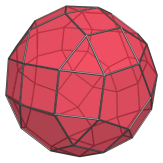J75 closely resembles the uniform rhombicosidodecahedron, but, just like J74, it can be recognized by noting the tell-tale pairs of adjacent squares and strips of 3 squares, as well as triangles that share an edge with a pentagon: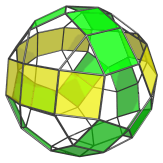Most notably, the strips of 3 adjacent squares in J75 (shown in yellow in the above image) are a distinctive feature of the metabigyrates and trigyrate of the rhombicosidodecahedron. There are 3 such strips in J75, and 9 pairs of adjacent squares.

The uniform rhombicosidodecahedron does not have square faces that share edges with each other, nor does it have triangles that share edges with pentagons. These features arise from the gyrated configuration of the 3 pentagonal cupola segments highlighted below:## Projections

Here are some views of the trigyrate rhombicosidodecahedron from various angles:

Projection Description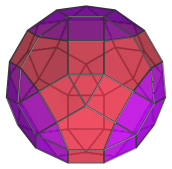Top view.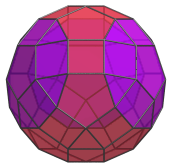Front view, parallel to pair of opposite triangles.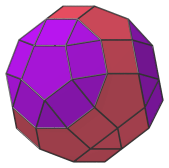Side view.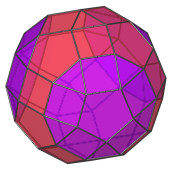Oblique view.

## Coordinates

The Cartesian coordinates of the trigyrate rhombicosidodecahedron with edge length 2 are:

• (0, ±(φ+2), φ2)
• (0, (φ+2), −φ2)
• (±φ2, 0, −(φ+2))
• (±(φ+2), ±φ2, 0)
• (±1, ±1, φ3)
• (±1, 1, −φ3)
• (±1, ±φ3, ±1)
• (±φ3, ±1, −1)
• (±2φ, ±φ, −φ2)
• (±φ2, ±2φ, ±φ)
• (±φ, ±φ2, 2φ)
• (±φ, φ2, −2φ)
• (±(8φ+1)/5, ±1, (6φ+7)/5)
• (±(8φ+6)/5, ±φ, (7+φ)/5)
• (±(13φ+1)/5, 0, (φ+2)/5)
• (±1, −(6φ+7)/5, −(8φ+1)/5)
• (±φ, −(7+φ)/5, −(8φ+6)/5)
• (0, −(φ+2)/5, −(13φ+1)/5)

where φ=(1+√5)/2 is the Golden Ratio.

Last updated 29 Apr 2019.